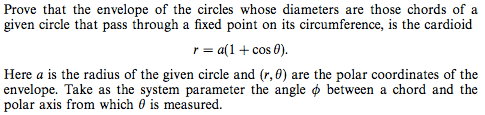# Show the envelope of circles around a circle is a cardioid

• I
Happiness
Is there a shorter way to get the answer, the polar equation of a cardioid, directly?My solution involves some tedious work and doesn't give the polar equation directly:

The equation of the member curves (circles) is

##f(x, y, \phi)=(x-a\cos^2\phi)^2+(y-a\cos\phi\sin\phi)^2-a^2\cos^2\phi=0##. --- (1)

To find the envelope curve, we impose ##\frac{\partial f}{\partial\phi}=0##. Thus, we have

##2\sin\phi\cos\phi u-(cos^2\phi-sin^2\phi)v+\sin\phi\cos\phi a=0\,\,\,##, where ##u=x-a\cos^2\phi\,\,\,##and ##\,\,\,v=y-a\cos\phi\sin\phi##.

Making ##v## the subject and then substituting into equation (1), we have (after some tedious work)

##x=2a\cos^2\phi\cos2\phi##.

Making ##u## the subject and then substituting into equation (1), we have (after some tedious work)

##y=2a\cos^2\phi\sin2\phi##.

Last edited:

Hey Happiness.

Where are the "variables" u and v in your initial function?

Happiness
Hey Happiness.

Where are the "variables" u and v in your initial function?

Hey chiro

##u=x-a\cos^2\phi\,\,\,##and ##\,\,\,v=y-a\cos\phi\sin\phi##

The two equations are

$$(x-a\cos^2\phi)^2+(y-a\cos\phi\sin\phi)^2-a^2\cos^2\phi=0$$

$$2\sin\phi\cos\phi(x-a\cos^2\phi)-(\cos^2\phi-\sin^2\phi)(y-a\cos\phi\sin\phi)+\sin\phi\cos\phi a=0$$

Is there a shorter way to convert this pair of equation into the polar equation ##\rho=a(1+\cos\theta)##?

I introduced ##u## and ##v## just to make them look shorter:

$$u^2+v^2-a^2\cos^2\phi=0$$

$$2\sin\phi\cos\phi u-(\cos^2\phi-\sin^2\phi)v+\sin\phi\cos\phi a=0$$

Last edited:
I'd recommend unrolling all of your variables so that you only get a function of independent ones since you can potentially miss things with the chain rule (of derivatives).

Since you have two relations (one is a function, the other possibly a relation) you will need to tell us if you are trying to optimize the function with regard to the constraint only (which seems to be the case but needs clarification) or whether you are trying to optimize a multi-variable function (which in this case would be using both functions and then trying to optimize a second one in terms of a derivative in conjunction with the first).

If it's the first then you will need to probably use a normal Jacobian and Hessian formulation to get the critical points as well as something like Lagrange multipliers.

If it is the second then you either have to do a bi-variate optimization problem or find a way to translate one to the other (like with your u and v analogy) and do the first.

Happiness
I'd recommend unrolling all of your variables so that you only get a function of independent ones since you can potentially miss things with the chain rule (of derivatives).

Since you have two relations (one is a function, the other possibly a relation) you will need to tell us if you are trying to optimize the function with regard to the constraint only (which seems to be the case but needs clarification) or whether you are trying to optimize a multi-variable function (which in this case would be using both functions and then trying to optimize a second one in terms of a derivative in conjunction with the first).

If it's the first then you will need to probably use a normal Jacobian and Hessian formulation to get the critical points as well as something like Lagrange multipliers.

If it is the second then you either have to do a bi-variate optimization problem or find a way to translate one to the other (like with your u and v analogy) and do the first.

The two equations are

$$(x-a\cos^2\phi)^2+(y-a\cos\phi\sin\phi)^2-a^2\cos^2\phi=0 -(1)$$

$$2\sin\phi\cos\phi(x-a\cos^2\phi)-(\cos^2\phi-\sin^2\phi)(y-a\cos\phi\sin\phi)+\sin\phi\cos\phi a=0 -(2)$$

Equation (2) is obtained from equation (1) by differentiating wrt ##\phi##. This part is easy.

The troublesome part is to show that these two equations together is equivalent to the polar equation ##r=a(1+\cos\theta)##. I get the answer in the end but my method involves laborious work of completing the square and scarily arduous work of converting the trigonometric functions raised to different powers into various forms using several identities in the hope that they will all simplify into a perfect square. The manipulation of the trigonometric functions is the difficult part because I do not know which form they should be converted to.

That's mainly a conversion of basis problem and that is known (given existing mathematical methods) to be difficult in general and it's the reason why differential geometry is not only hard but not that developed yet (it's a big generalization in comparison to the well developed framework of linear algebra).

What you are looking to do if I read your reply correctly is find some kind of technique to do an arbitrary change of basis.

Unfortunately the way current mathematics is setup, most of these results require "tricks" and "guesses" (like the one you use) and the typical co-ordinate systems/bases are often very restrictive.

The only thing I would recommend is taking a look at tensor analysis and differential geometry and go from spherical polar to another system and show the inverse relation holds with respect to the two bases. I'm pretty sure you did something similar but in applied mathematics and engineering, going from one space to another using matrix techniques is something these practitioners have to do and there are techniques to "invert" the basis given how the space "curves" as a function of variables.

The linear case is special in that it's derivatives are always zero meaning the vectors stay the same direction and span a normal linear plane.

If the above sounds like "gobbledy-gook" then pick up a book on tensor analysis (preferably an engineering related one since it will be more applied) and look at how to change the basis of the space with derivatives and tensor objects.